# Experimental quantum compressed sensing for a seven-qubit system

## Abstract

Well-controlled quantum devices with their increasing system size face a new roadblock hindering further development of quantum technologies. The effort of quantum tomography—the reconstruction of states and processes of a quantum device—scales unfavourably: state-of-the-art systems can no longer be characterized. Quantum compressed sensing mitigates this problem by reconstructing states from incomplete data. Here we present an experimental implementation of compressed tomography of a seven-qubit system—a topological colour code prepared in a trapped ion architecture. We are in the highly incomplete—127 Pauli basis measurement settings—and highly noisy—100 repetitions each—regime. Originally, compressed sensing was advocated for states with few non-zero eigenvalues. We argue that low-rank estimates are appropriate in general since statistical noise enables reliable reconstruction of only the leading eigenvectors. The remaining eigenvectors behave consistently with a random-matrix model that carries no information about the true state.

## Introduction

Recent years have seen rapid progress in the development of quantum technologies, with precisely controlled quantum systems reaching ever larger system sizes. Specifically, for systems of trapped ions, arrays of tens or more individual ions have been engineered and manipulated in their quantum state1,2,3,4, while architectures such as superconducting qubits5,6 and neutral atoms7,8, among many others, are also developing rapidly. These technological and scientific developments have enabled implementations of small-scale quantum simulators2,3,4, small measurement-based quantum computations9, proof-of-principle gate-based quantum computations1,10,11,12 and quantum error correction, for example, based on topological colour codes1.

As a result of this fast development, a new roadblock is of increasing concern: the fact that the Hilbert space dimension scales exponentially means that traditional methods for the experimental characterization of quantum states and processes become infeasible even for intermediate system sizes. This is problematic since such systems are the building blocks for emerging quantum technologies. To mitigate this problem, it has been suggested to use various structural properties of natural quantum systems—for example, high purity, symmetries, sparsity in a known basis or entanglement area laws—to reduce the effort of characterization13,14,15,16,17,18,19. For the purposes of this work, we refer to a quantum system as being intermediate-sized if it has five to ten physical qubits. This is the range where quantum error correction of one- and two-qubit logical gates becomes possible and full-state reconstruction methods are most useful.

Here we are relying on the technique of ‘compressed sensing’, which has emerged over the past decade in the field of classical data analysis20,21. It is now routinely used to estimate vectors or matrices from incomplete information, with applications in such diverse fields as image processing, seismology, wireless communication and many more21,22. Furthermore, compressed sensing for low-rank matrices has been adapted as a tool for quantum system characterization—also referred to as quantum tomography—in a series of works13,15,23. A particularly appealing feature of ‘quantum compressed sensing’ (the use of compressed sensing for quantum tomography) is the fact that there is no need to make any a priori assumptions about the true quantum state15,24 since the validity of the reconstruction can be subsequently verified.

Quantum compressed sensing is most effective on density matrices with quickly decaying eigenvalues. Such a state can be well approximated by a matrix of rank r (much smaller than the dimension d of the Hilbert space) and depends only on O(rd) parameters—significantly fewer than the d2 parameters required in general. In quantum information experiments, the goal is often to prepare a pure state, described by a rank-1 density matrix. Noise effects will typically require one to include more than one eigenvalue to obtain a good approximation of the true state. However, in highly controlled experiments, the number of additional eigenvalues is expected to be small. In this context, the theory of compressed sensing showed for the first time that the reduced number of parameters is reflected in a reduced effort in both measurements and computation required for tomographic reconstruction. Indeed, it has been rigorously proven that an (approximate) rank-r density matrix can be recovered from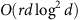experimentally measured parameters13. This performance—close to the absolute lower bound of O(rd)—can even be achieved when the eigenbasis is completely unknown13.

In this work, we demonstrate that this approach is reaching maturity by implementing an experimental reconstruction of the state of a seven-qubit system from an informationally incomplete set of measurements. In fact, we report the first implementation of compressed state reconstruction on a platform of seven trapped ions, which are prepared in a state of a topological colour code25. Important steps towards quantum compressed sensing protocols have been implemented17,26,27. In particular, previous work27 has explored quantum state tomography based on expectation values using techniques of compressed sensing in a six-qubit photonic system. Here we continue to push towards much bigger systems by developing more scalable compressed sensing reconstruction tools. In addition, we have used a variety of computationally efficient estimators to achieve recovery in practice, which we revisit when we describe the numerical techniques used in our experiment.

A secondary objective of this work is to argue that compressed tomography, while originally developed for density matrices with a small number of dominating eigenvalues, can also be appropriate in situations where the unknown true density matrix is not, in fact, of low rank. This counterintuitive conclusion follows from the finding that in realistic regimes (for example, incomplete data), the statistical signal-to-noise ratio is such that only the leading eigenvectors of the density matrix can be reliably reconstructed. Indeed, we find that the tail of least-significant eigenvectors behaves in ways consistent with a random-matrix model, which means that reporting more than the first few eigenvectors reveals no information about the true state and thus amounts to overfitting. To make this insight more concrete, we formulate a task that is reminiscent of support identification in compressed sensing—the problem of deciding which eigenspaces should be included in an estimate (see, for example, ref. 28), which we call ‘quantum support identification’. We give heuristics for identifying the relevant support, based on comparing the behaviour of the estimate with a random-matrix model. Our findings are consistent with a recent approach that recommends spectral thresholding for statistical reasons29; and another that shows that statistical noise in state reconstruction protocols can manifest itself by giving rise to random-matrix-like behaviour of the spectrum of the recovered state30.

Finally, we observe that the estimators introduced in the context of compressed sensing reconstruct the leading eigenvectors more faithfully than more traditional approaches, at the price of being less faithful to the spectral tail. This suggests that one should employ the former if one is more interested in learning about ‘coherent errors’ (that is, the way in which the first eigenvector deviates from its target), while the latter are better-suited to analyse ‘incoherent noise processes’ that drive up the rank.

## Results

### Physical system and data model

We begin by explaining the physical architecture of trapped ions that serves as the platform for this endeavour. In the considered ion-trap quantum computer, 40Ca+ ions are stored in a linear Paul trap. Each physical qubit is encoded inand the metastable, excited state corresponding to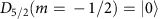. Manipulation of the qubit is performed by laser pulses resonant (or close to resonant) to the atomic transitions of 40Ca+. The universal set of quantum gates is implemented using three types of operations: collective operations of the form exp(−i(θ/2)Sφ) with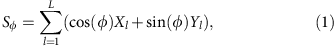and entangling operations of the form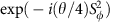, reflecting the entangling Mølmer–Sørenson interaction31. Here Xl, Yl and Zl are the Pauli operators of qubit l, θt is determined by the Rabi frequency Ω and laser pulse duration t>0, and φ is determined by the relative phase between qubit and laser. The third type of operations is generated by single-qubit phase rotations induced by localized AC-Stark shifts. More details of this experimental set-up are covered in ref. 32.

Within this experimental setting involving L=7 qubits, quantum states have been prepared to the best of the experimental knowledge that, however, is limited by statistical noise and systematic errors. The quantum states are described mathematically by density operators ρHd(C) (Hermitian d × d matrices) for d=2L that satisfy tr(ρ)=1 and ρ≥0. In all of the experiments, the aim was to prepare a pure state vector contained in the code space, which is a two-dimensional subspace of the Hilbert space of seven qubits spanned by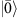and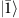. Here the state vectorsandspan the code space and are joint +1 eigenstates of the set of stabilizer operators that define the code. The stabilizer operators are given explicitly in ref. 1. The particular basis for the code space is chosen by pickingandto be the eigenvectors of Z1 ZL with eigenvalues +1 and −1, respectively. The states that the ideal experiment would prepare will be referred to as ‘anticipated states’ in what follows. Bothandare code words of a Calderbank–Shor–Steane code33,34 originating from the theory of quantum error correction designed to protect fragile quantum information against unwanted local noise. At the same time, they can be seen as the smallest fully functional instances of a ‘topological colour code’25, which are topological quantum error-correcting codes defined on physical systems supported on two-dimensional lattices.

For each state, a set of n=127 Pauli basis ‘measurement settings’ is chosen. (An informationally complete set would contain 3L=2,187 settings.) Each measurement setting j is characterized by a choice of a local Pauli matrix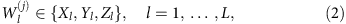for each of the L qubits. The lth qubit is measured in the eigenbasis of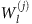. There are two possible outcomes for each qubit, and therefore a total of 2L possible outcomes per experiment. Each specific outcome k is associated with a projection operatorwhere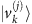is a tensor product of eigenvectors of the.

For each measurement setting, the measurement is repeated m=100 times and the statistics of measurement outcomes is recorded. From the relative frequencies of outcomes k, the probability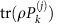is estimated. Note that because of the relatively small number of repetitions of the measurements per setting, given 2L potential outcomes, many of the possible outcomes will not appear even once. This implies that we have a highly noisy signal.

Let us denote the measurement settings that have been chosen as VW, where W is the set of all possible measurement settings. We define the ‘sampling operator’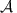: Hd(C)→nd as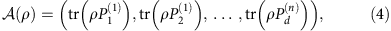with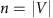the number of chosen settings. That is, the sampling operator is the linear map that simply returns the list of expectation values of the observablesmeasured in the state ρ. The data taken are of the type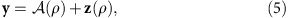where the zero-mean random vector z(ρ) captures the statistical noise. The outcomes for any given basis follow a multinomial distribution, from which one obtains the expression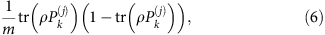for the second moment of each given component of y.

For completeness, we note that the ‘Pauli basis measurements’ considered here differ from the ‘Pauli correlation measurements’ that were the basis of previous works on quantum compressed sensing13. Pauli correlation measurements are of the form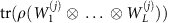, where again theare Pauli matrices acting on the lth qubit. These correlators associate ‘one’ expectation value with each choice of local Pauli matrices and appear, for example, as syndrome measurements in quantum error correction. As detailed above, the ‘basis measurements’ yield 2L parameters per choice of local Pauli matrices. This is the number of ways of picking one of the two eigenvectors of each Pauli matrix. Basis measurements, which thus give much more detailed information per setting, appear naturally in the ion-trap architecture used in this work. Moreover, one can recover Pauli correlations from basis measurements via the relationship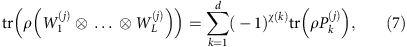where χ(k) denotes the parity of the binary representation of the integer k.

### Estimators and state reconstruction

In statistics, an ‘estimator’ is a rule for mapping observed data (here, outcomes y) to an estimate for an unknown quantity (here, a density matrix ρ). At the heart of the discussion is an estimator that is particularly common in the compressed sensing literature. This is the so-called ‘trace norm minimizer’ referred here to as TNM: this is an estimator based on trace minimization with a positivity constraint. We solve the following problemwhere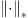is the nuclear or matrix trace norm and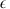>0 is the error level. The trace norm can be shown to be the tightest convex relaxation of rank. Thus, the regularization term both encourages low-rank solutions and, due to convexity, can be minimized efficiently21,23. This estimator resembles the Dantzig selector15. It is an estimator based on the intuition derived from compressed sensing that under the restricted isometry property35, the positive semidefinite trace norm minimizer compatible with the data is the actual state13. This estimator can be cast as a semidefinite program (SDP), which means that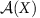has to be computed in matrix form, with all memory requirements that come along with it. For L=7 qubits, as presented in this work, using a SDP solver is still feasible since the number of measurement settings is not too large (about <1,000 on a standard workstation). However, better-scaling algorithms exist for this problem and can readily be used. Note that for this estimator, one has to estimate the error-level parameter>0 (ref. 15). In practice, one can recognize two limiting regimes for choosing this parameter: ifis too small, the optimization problem is infeasible, that is, there is no solution X that satisfies the constraints. Ifis too big, in contrast, one finds a solution that it is biased towards low-rank states, that is, solution X is close to being pure. Here we work in the former regime, that is, we use the smallestparameter for which the optimization problem converges.

To complement our study, in addition to the TNM estimator, we use a simple and versatile estimator based on the least squares method, which we refer to as LS. It is a LS estimator with positivity constraint and it solves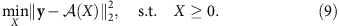The positivity constraint on X helps the estimation process, based on the intuition that the set of feasible density operators lies at the intersection of those operators compatible with the data and the positive cone. In practice, one can perform very good estimation with this estimator, even with informationally incomplete measurements, if the actual state is not too mixed and hence close to the boundary of state space. There is empirical evidence for this observation, which can also be made precise36. In fact, the additional positivity constraint in this type of problems renders essentially all estimators equivalent37.

Formally, both estimators can be realized via algorithms that are efficient in the sense that they can be solved in polynomial time in the size of the input. In fact, they are specifically suited to the regime of intermediate/large quantum systems and comparably little data in which we are interested. However, since our analysis involves hundreds of estimation problems, additional theoretical efforts are required to arrive at an implementation that performs well in practice in the regime of intermediate and large quantum systems for the LS estimator. To achieve this, we here introduce a new specific implementation for LS estimator. In this approach, we parametrize the quantum state ρ as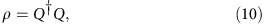for some r × d complex matrix Q, where r controls the rank of ρ. We then considerwhereis the vector 2 norm.

Using this parameterization of ρ ensures that it is positive semidefinite by construction. The optimization problem itself is then solved using a gradient method. A gradient flow on the basis of ρ directly would introduce negative eigenvalues in every step. In contrast, we optimize over Q, using the fact that we can analytically compute the gradient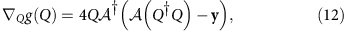of the objective function in equation (11). This way, we dispense with the unnecessary and computationally expensive projection step that would otherwise be needed to enforce positivity.

This simplification significantly improves the computational effort as compared to earlier estimators that made use of an iterative gradient method based on ρ. We refer to this gradient method based on a manifestly positive parametrization of states as GRAD. We present details of this algorithm in the methods section. This improvement is of great relevance for the study we carry out in the next section.

### Quantum support identification

The traditional goal of quantum state tomography is to estimate the true density matrix of the system—that is, the one that would result in the limit of infinitely many measurements, when all statistical uncertainties have vanished (assuming no drift or other systematic errors). We will now argue that in a high-dimensional setting, with limited data, it may be neither possible nor desirable to obtain a complete estimate of the true state.

It is not necessarily desirable, because it is unclear that a high-dimensional matrix would provide either interpretable or actionable information. Consider a typical use case for tomography, where the difference between the anticipated state and the leading eigenvectors encodes useful information about the dominating error sources. The eigenvectors associated with the first few eigenvalues contain the most useful information about noise effects, and based in these inputs an experimentalist can adjust the apparatus to achieve a higher fidelity in future runs. However, it is unclear which action would possibly follow from knowing, say, the exact form of the 100th eigenvector.

At the same time, the data obtained may also not be sufficient to estimate all the parameters of the full density matrix to a sensible accuracy. Indeed, trying to fit too many degrees of freedom to noisy data results in ‘overfitting’, where the estimate depends strongly on statistical fluctuations and only to a small degree on the true state. To combat this, ‘model selection’ methods give rules for selecting a lower-dimensional model if the amount and variability of the data do not allow for a reconstruction of the full set of unknown parameters38.

In the context of quantum state estimation, ‘spectral thresholding’ has been proposed as a model selection method and theoretically analysed in the regime of informationally complete measurements29. Spectral thresholding here means that a lower-dimensional model is selected by setting all eigenvalues of the estimate to zero if they are below a threshold value that depends on the dimension of the Hilbert space and the variance of the individual measurements29.

Here we propose a new heuristic for selecting which eigenvalues of an estimate to keep and which to discard as not meaningful. While it lacks the rigorous guarantees of ref. 29, it is applicable in more general situations. It is based on a transparent criterion: parameters of an estimated density matrix should not be reported if they behave in ways consistent with a random-matrix model—that is, if they can be explained as resulting from a purely random noise without any signal. The use of random-matrix theory for the purpose of testing the significance of estimated small eigenvalues has recently been discussed in ref. 30. While related to our approach, the goals and methods are distinctly different: the aim of ref. 30 is to make statements about the true rank of an unknown density operator by looking at the properties of its spectrum. Here, in contrast, we want to identify the part of the estimate we can already trust given the data available while looking at the properties of the random eigenvectors of the reconstructed density matrix. Our approach, in addition, does estimate the correct true rank when enough information is provided. See Supplementary Discussion for a detailed description.

Technically, for a given data set y(1), the spectral decomposition of the positive semidefinite estimate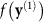can be written as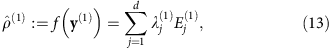with decreasingly ordered eigenvalues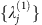and corresponding eigenprojections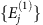, where f denotes the action of the estimator on the data. When insufficient data are taken in an experiment, not all eigenprojections can be characterized equally well. Only for some eigenprojections will one have provided sufficient data. They, concomitantly, will have low uncertainties and thus will be common to different estimates of the same state based on different realizations of the experiment, while the other directions will fluctuate wildly based on the particular data obtained. Generating a different data set y(2) using the bootstrapping techniques detailed below, we arrive at the estimate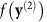with decomposition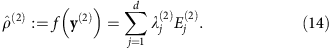Our figure of merit is based on the Hilbert–Schmidt scalar product of the eigenprojections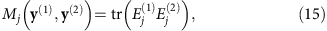where j=1, …, d. In the informationally incomplete regime we are in, this quantity will show a strong overlap only between the dominant eigenvectors. For the eigenvectors of the complement, the overlaps resemble the overlap of state vectors chosen randomly from the unitarily invariant Haar measure. In the light of this, the spectral thresholding parameter k is taken to be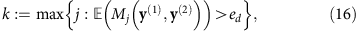in expectation over pairs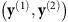, where the threshold ed is chosen asfor the random variable defined as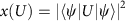as overlaps between Haar random state vectors from Cd, where U is a Haar random unitary. Specifically, a random-matrix theory computation (see methods) gives,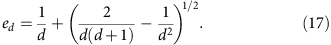On the basis of such a significance threshold, for the estimate based on the data, we return the spectrally thresholded state ρk with a normalization c>0, where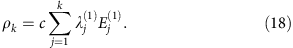Let us now define the protocol we follow to provide an estimate that has low enough rank to be compatible with few data and yet avoid overfitting. For this, we review the concept of bootstrapping. We consider two types of bootstrapping: parametric and non-parametric. In parametric bootstrapping, from the reconstructed density matrix, one simulates the experimental measurements (sampled according to the appropriate noise statistics) and for each sample data realization one computes a new estimated density matrix. In non-parametric bootstrapping, however, the measured frequencies are assumed as the true probabilities, which in turn are used to simulate (sample) new data sets that are used, as before, to compute an ensemble of estimated density matrices. In both cases, one uses the ensemble of recovered density matrices to gain confidence on the reconstructed state.

The way we proceed is the following: from the experimentally measured frequencies, we do either parametric or non-parametric bootstrapping to generate an ensemble of estimated density matrices using the GRAD algorithm for the LS estimator. We do it in this way because this particular implementation is able to deal with hundreds of bootstrapped data sets in a reasonably short time. Then, we find their spectral decomposition and order their eigenvalues and eigenvectors in descending order as explained above. Subsequently, for all possible pairs of estimated density matrices, we compute the mean of equation (15) for all j. Finally, we report as the rank of the reconstructed state the largest j, equation (16), for which the quantity Mj has an overlap greater than the threshold computed in equation (17). The results are shown in Fig. 1c and in Supplementary Discussion, where we also show the performance of this method via extensive numerical simulations.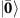Figure 1: Example of quantum state reconstruction for the logical state vector.

## Discussion

In each experiment we have performed, the ‘anticipated states’ were taken from the code space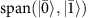of the topological colour code. In Fig. 1, we present a graphical representation of an instance of such a reconstruction (for more examples, please see Supplementary Discussion). It depicts the reconstructed state based on the TNM estimator, the one obtained via the LS estimator computed with our GRAD algorithm, and a truncated estimate that we call ‘spectral thresholding’ estimate, where only the highest eigenvalues have been kept via the procedure described in the previous section.

From Fig. 1, we see that the estimators give rise to valid and faithful reconstructions of the anticipated state, in that the reconstructed states are close in fidelity to the anticipated states, though some estimators report higher fidelities than others. The computational runtime for the estimators is moreover quite modest, of the order of an hour, and of a few minutes for the GRAD estimator. This analysis, Fig. 1a, can be seen as a first experimental implementation of quantum state tomography based on a compressed sensing methodology for high-dimensional quantum systems.

If we compare a typical figure of merit for the quality of a state reconstruction—the fidelity to the anticipated state—then we notice that the three reported reconstruction fidelities differ from F=0.43 (TNM estimate) down to F=0.30 (LS estimate) on the same data. We hypothesize that this difference is due to a combination of limited data (applicable to all estimators) and the fact that the TNM estimate gives a much higher penalty to mixed states than the other two estimators. In fact, we empirically observe that the purity of the reconstructed state strongly depends on the choice of error-level parameter. For largevalues, the TNM estimator will favour reconstructing nearly pure states, and thus it will not estimate the tail of the spectrum when data are scarce. Because of this, it is expected to be better at diagnosing ‘coherent’ errors, as it will return the dominant pure state. By contrast, for smallvalues or when other estimators are used, one sacrifices purity to better match the spectrum, which causes the reconstructed state to have a poorer fidelity with the anticipated state. Thus, these estimators might be better for diagnosing ‘incoherent’ noise, as these methods retain visibility for excessive noise in the system. In the context of process tomography of near unitary maps, a similar effect has been observed39.

We can gather evidence for our hypothesis by looking at the diagonal matrix elements of the reconstructed states in the anticipated basis, meaning the stabilizer basis that includes the anticipated state. In Fig. 2a,b, we see the absolute values of the matrix elements of the reconstructed states using this basis. Here it is much clearer that the TNM estimate with largeparameter is detecting coherent noise, while the LS estimate (and the spectral thresholding estimate, not shown) achieve a more mixed reconstruction. In fact, Fig. 2c shows that in every case the majority of the diagonal elements are decaying exponentially when ordered in decreasing magnitude, but with a much more rapid initial decay for the TNM estimator with large. Although this constitutes evidence for our hypothesis, much more work should be done to determine whether there is any advantage to using different estimators to highlight different features of the noise.Figure 2: Coherent versus incoherent error analysis for the logical state vector.

In this context, from our analysis of the experimental data at hand, and given the amount of available data, we see evidence that the errors present in our experiment are mostly incoherent. The absence of strong coherences (off-diagonal elements) in the reconstructed density matrix (Fig. 2a; Supplementary Fig. 3) seems to indicate that. Our methods demonstrate that the principal components of the density matrix have a large overlap with the anticipated state. Notice that observing this feature in such a large Hilbert space by luck is nearly impossible. This observation gives further actionable advice from the tomographic analysis, in that an improvement of the preparation should aim at removing such incoherent errors.

As stated earlier, the purpose of our work is twofold. On one hand, it presents a successful first compressed sensing tomography implementation on a seven-qubit quantum experiment, using estimators and reconstruction techniques that are efficient in the Hilbert space dimension. In this way, it demonstrates the potential of using the machinery of the ‘big data’ paradigm to assess quantum systems close to the limit of what is experimentally feasible. On the other hand, more conceptually, we discuss ideas of ‘quantum support identification’, related to the question of what quantum state tomography can actually mean in the regime of informationally incomplete data for intermediately sized quantum systems. We advocate a paradigm that only those low-rank states should be reported that have a statistical basis and we see that the compressed sensing machinery, that is, TNM with minimal, is enough to give an estimate of such characteristics without being too expensive to compute. We also saw evidence that using a TNM estimate with largecan be useful to detect coherent errors.

Quantum tomography—the task of reconstructing unknown states from data—is a key primitive in quantum technologies. At its heart, it aims at providing actionable advice upon which the experimenter can make the appropriate modifications to an experimental set-up. It goes beyond mere certification of the correctness of an anticipated preparation of a quantum state15,16,40: by learning in what way the actually prepared state deviates from the anticipated one, one can modify the apparatus appropriately to improve performance in future runs. It is our hope that we can inspire further work on the certification and reconstruction of quantum states and processes for increasingly large quantum systems, overcoming the roadblock against further development in quantum technologies.

## Methods

Here we present details of the reconstruction sketched in the main text. In our approach, which we called GRAD, solving the LS estimator, we parametrize the density matrix aswhich makes it manifestly positive semidefinite. We then solvewith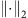being the vector 2 norm. We do this using a gradient search algorithm, but notably for Q and not for ρ itself. This is the key feature of this approach. This method derives from the idea presented in ref. 41. The basic iteration step in a sequence of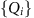is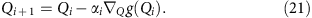For the moment αi>0 is chosen to be a sufficiently small step size, but this can surely be refined to a conjugate gradient method if absolutely necessary, and can hence be tuned to increase convergence speed. In our case, the actual gradient can be computed analytically. Note that g(Q) can be written as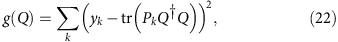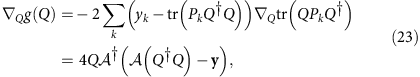as stated in the main text. Here we have used the standard matrix identity XTr(XBX)=XB+XB for the particular case in which B is Hermitian. We then iterate the previous equation until reaching convergence. The state is renormalized at the end, as the trace is not constrained in this way. This is an extremely fast and elegant way to incorporate positivity of QQ.

It is worth mentioning that this approach significantly improves earlier ideas deriving from refs 42, 43, in which a gradient method for the state ρ was combined with a suitable projection. Specifically,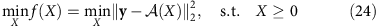was solved by moving away from the SDP and solving the optimization problem using a gradient search algorithm. The basic iteration is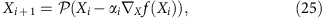where here X is the gradient operator with respect to matrix X and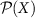is a projector that makes the estimated state positive semidefinite. The gradient is computed explicitly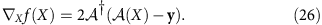While this approach also works, the projection significantly slows down the algorithm, hence the need for our method that directly incorporates positivity.

### The random-matrix model

Here we present results from random-matrix theory on expected overlaps of random vectors. Specifically, for an arbitrary vector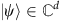, we consider the random variable defined by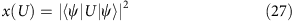and moments thereof with respect to the Haar measure. This quantity is easily identified as the overlap of two random vectors from Cd. We compute first and second moments thereof. They can be computed making use of the powerful Weingarten function formalism44. We find in terms of a Weingarten function Wg,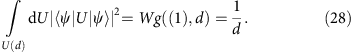The second moments can be expressed asusing suitable Weingarten functions. The sum over all permutations on two symbols in the relationship between Haar averages and Weingarten functions,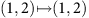and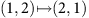, then simply gives rise to the above two terms. These result in the expression for the variance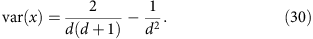In the main text, the quantity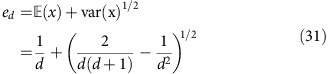has been derived from this.

### Data availability

The data that support the findings of this study are available from the corresponding author upon reasonable request.

How to cite this article: Riofrío, C. A. et al. Experimental quantum compressed sensing for a seven-qubit system. Nat. Commun. 8, 15305 doi: 10.1038/ncomms15305 (2017).

Publisher’s note: Springer Nature remains neutral with regard to jurisdictional claims in published maps and institutional affiliations.

## References

1. 1

Nigg, D. et al. Experimental quantum computations on a topologically encoded qubit. Science 345, 302–305 (2014).

2. 2

Lanyon, B. P. et al. Universal digital quantum simulation with trapped ions. Science 334, 57–61 (2011).

3. 3

Kaufmann, H. et al. Precise experimental investigation of eigenmodes in a planar ion crystal. Phys. Rev. Lett. 109, 263003 (2012).

4. 4

Blatt, R. & Roos, C. F. Quantum simulations with trapped ions. Nat. Phys. 8, 277–284 (2012).

5. 5

Barends, R. et al. Superconducting quantum circuits at the surface code threshold for fault tolerance. Nature 508, 500–5003 (2014).

6. 6

Ofek, N. et al. Extending the lifetime of a quantum bit with error correction in superconducting circuits. Nature 536, 441–445 (2016).

7. 7

Maller, K. M. et al. Rydberg-blockade controlled-not gate and entanglement in a two-dimensional array of neutral-atom qubits. Phys. Rev. A 92, 022336 (2015).

8. 8

Nogrette, F. et al. Single-atom trapping in holographic 2d arrays of microtraps with arbitrary geometries. Phys. Rev. X 4, 021034 (2014).

9. 9

Lanyon, B. P. et al. Measurement-based quantum computation with trapped ions. Phys. Rev. Lett. 111, 210501 (2013).

10. 10

Vandersypen, L. M. K. et al. Experimental realization of shor’s quantum factoring algorithm using nuclear magnetic resonance. Nature 414, 883–887 (2001).

11. 11

Fedorov, A., Steffen, L., Baur, M., da Silva, M. P. & Wallraff, A. Implementation of a Toffoli gate with superconducting circuits. Nature 481, 170–172 (2012).

12. 12

Gulde, S. et al. Implementation of the Deutsch-Jozsa algorithm on an ion-trap quantum computer. Nature 421, 48–50 (2003).

13. 13

Gross, D., Liu, Y.-K., Flammia, S. T., Becker, S. & Eisert, J. Quantum State Tomography via Compressed Sensing. Phys. Rev. Lett. 105, 150401 (2010).

14. 14

Cramer, M. et al. Efficient quantum state tomography. Nat. Commun. 1, 149 (2010).

15. 15

Flammia, S. T., Gross, D., Liu, Y.-K. & Eisert, J. Quantum tomography via compressed sensing: error bounds, sample complexity and efficient estimators. New J. Phys. 14, 095022 (2012).

16. 16

Flammia, S. T. & Liu, Y.-K. Direct Fidelity Estimation from Few Pauli Measurements. Phys. Rev. Lett. 106, 230501 (2011).

17. 17

Shabani, A. et al. Efficient measurement of quantum dynamics via compressive sensing. Phys. Rev. Lett. 106, 100401 (2011).

18. 18

Hübener, R., Mari, A. & Eisert, J. Wick’s theorem for matrix product states. Phys. Rev. Lett. 110, 040401 (2013).

19. 19

Steffens, A. et al. Towards experimental quantum field tomography with ultracold atoms. Nat. Commmun. 6, 7663 (2015).

20. 20

Candes, E. & Wakin, M. An introduction to compressive sampling. IEEE Signal Process. Mag. 25, 21–30 (2008).

21. 21

Foucart, S. & Rauhut, H. A Mathematical Introduction to Compressive Sensing Springer (2013).

22. 22

Eldar, Y. Compressed Sensing: Theory and Applications Cambridge Univ. Press (2012).

23. 23

Kueng, R., Rauhut, H. & Terstiege, U. Low rank matrix recovery from rank one measurements. Appl. Comp. Harmon. Anal. 42, 88–116 (2017).

24. 24

Carpentier, A., Eisert, J., Gross, D. & Nickl, R. Uncertainty quantification for matrix compressed sensing and quantum tomography problems. Preprint available at arXiv:1504.03234v2 (2015).

25. 25

Bombin, H. & Martin-Delgado, M. A. Topological quantum distillation. Phys. Rev. Lett. 97, 180501 (2006).

26. 26

Rodionov, A. V. et al. Compressed sensing quantum process tomography for superconducting quantum gates. Phys. Rev. B 90, 144504 (2014).

27. 27

Schwemmer, C. et al. Experimental comparison of efficient tomography schemes for a six-qubit state. Phys. Rev. Lett. 113, 040503 (2014).

28. 28

Waters, A. E., Sankaranarayanan, A. C. & Baraniuk, R. in Adv. Neur. Inf. Proc. Sys. (eds Shawe-Taylor, J., Zemel, R. S., Bartlett, P. L., Pereira, F. & Weinberger, K. Q.) Vol. 24, 1089–1097 (Curran Associates, Inc., 2011).

29. 29

Guta, M., Kypraios, T. & Dryden, I. Rank-based model selection for multiple ions quantum tomography. New J. Phys. 14, 105002 (2012).

30. 30

Knips, L. et al. How long does it take to obtain a physical density matrix? Preprint available at arXiv:1512.06866v1 (2015).

31. 31

Sørensen, A. & Mølmer, K. Quantum computation with ions in thermal motion. Phys. Rev. Lett. 82, 1971–1974 (1999).

32. 32

Schindler, P. et al. A quantum information processor with trapped ions. New J. Phys. 15, 123012 (2013).

33. 33

Calderbank, A. R. & Shor, P. W. Good quantum error-correcting codes exist. Phys. Rev. A 54, 1098–1105 (1996).

34. 34

Steane, A. M. Error correcting codes in quantum theory. Phys. Rev. Lett. 77, 793–797 (1996).

35. 35

Candes, E. J. The restricted isometry property and its implications for compressed sensing. C. R. Acad. Sci. 346, 589–592 (2008).

36. 36

Suess, D., Rudnicki, L. & Gross, D. Error regions in quantum state tomography: computational complexity caused by geometry of quantum states. Preprint available at ArXiv:1608.00374 (2016).

37. 37

Kalev, A., Kosut, R. L. & Deutsch, I. H. Quantum tomography protocols with positivity are compressed sensing protocols. njp Quant. Inf. 1, 15018 (2015).

38. 38

Akaike, H. A new look at the statistical model identification. IEEE Trans. Aut. Contr. 19, 716 (1974).

39. 39

Baldwin, C. H., Kalev, A. & Deutsch, I. H. Quantum process tomography of unitary and near-unitary maps. Phys. Rev. A 90, 012110 (2014).

40. 40

Aolita, L., Gogolin, C., Kliesch, M. & Eisert, J. Reliable quantum certification for photonic quantum technologies. Nat. Commun. 6, 8498 (2015).

41. 41

Burer, S. & Monteiro, R. D. C. A nonlinear programming algorithm for solving semidefinite programs via low-rank factorisation. Math. Program. 95, 329 (2003).

42. 42

Kyrillidis, A., Becker, S., Cevher, V. & Koch, C. Sparse projections onto the simplex. Preprint available at arXiv:1206.1529 (2012).

43. 43

Becker, S., Cevher, V. & Kyrillidis, A. Randomized low-memory singular value projection. Preprint available at arXiv:1303.0167 (2013).

44. 44

Weingarten, D. Asymptotic behavior of group integrals in the limit of infinite rank. J. Math. Phys. 19, 999–1001 (1978).

## Acknowledgements

We thank A. Steffens, M. Kliesch and C. Ferrie for discussions and comments on the manuscript. This work has been supported by the Templeton Foundation, the EU (RAQUEL, AQuS), the ERC (TAQ), by the Freie Universität Berlin and the University of Cologne within the Excellence Initiative of the German Federal and State Governments, the DFG (SPP 1798 CoSIP, EI 519/7-1, EI 519/9-1, CRC 183 and GRO 4334/2-1), by the Austrian Science Fund (FWF) through the SFB FoQus (FWF Project No. F4002-N16), the Institut für Quanteninformation GmbH, the BMBF (Q.com), as well as by Universities Australia and DAAD’s Joint Research Co-operation Scheme (using funds provided by the German Federal Ministry of Education and Research). This work was also supported by the Australian Research Council via EQuS project number CE11001013, and by the US Army Research Office under the grants W911NF-14-1-0098, W911NF-16-1-0070 and W911NF-14-1-0103 within the QCVV program. Furthermore, this research was funded by the Office of the Director of National Intelligence (ODNI), Intelligence Advanced Research Projects Activity (IARPA), through the Army Research Office grant W911NF-10-1-0284. All statements of fact, opinion or conclusions contained herein are those of the authors and should not be construed as representing the official views or policies of IARPA, the ODNI or the US Government. S.T.F. also acknowledges support from an Australian Research Council Future Fellowship FT130101744.

## Author information

Authors

### Contributions

C.A.R., D.G., S.T.F. and J.E. developed the theoretical framework. T.M., D.N. and R.B. designed and performed the experiment. C.A.R. carried out the data analysis and simulations. All authors contributed to the writing of the manuscript.

### Corresponding authors

Correspondence to C. A. Riofrío or J. Eisert.

## Ethics declarations

### Competing interests

The authors declare no competing financial interests.

## Supplementary information

### Supplementary Information

Supplementary figures, supplementary discussion and supplementary references. (PDF 5049 kb)

## Rights and permissions

Reprints and Permissions

• ### Fast state tomography with optimal error bounds

• M Guţă
• , J Kahn
• , R Kueng
•  & J A Tropp

Journal of Physics A: Mathematical and Theoretical (2020)

• ### Complete Quantum-State Tomography with a Local Random Field

• Pengcheng Yang
• , Min Yu
• , Ralf Betzholz
• , Christian Arenz
•  & Jianming Cai

Physical Review Letters (2020)

• ### Non-Markovian Noise Characterization with the Transfer Tensor Method

• Yu-Qin Chen
• , Kai-Li Ma
• , Yi-Cong Zheng
• , Jonathan Allcock
• , Shengyu Zhang
•  & Chang-Yu Hsieh

Physical Review Applied (2020)

• ### Quantum State Filter With Disturbance and Noise

• Jiaojiao Zhang
• , Shuang Cong
• , Qing Ling
• , Kezhi Li
•  & Herschel Rabitz

IEEE Transactions on Automatic Control (2020)

• ### Design and experimental performance of local entanglement witness operators

• David Amaro
•  & Markus Müller

Physical Review A (2020)